# Quiz 13: Pediatric and Adult Dosages Based on Body Weight

Medicine

Equivalent 1g = 1,000 mg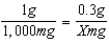X = 1,000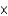0.3 X = 300 mg X capsules =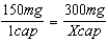150X = 300 X = 2 capsules

X tablets =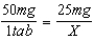50X = 25 X =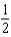tablet

X mL =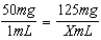50X = 125 X = 2.5 mL